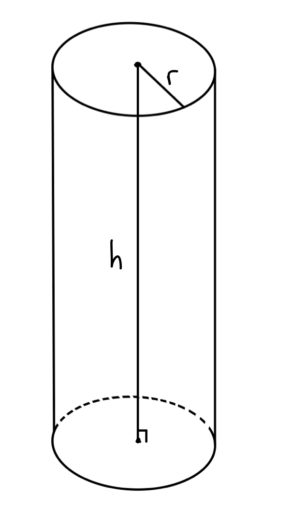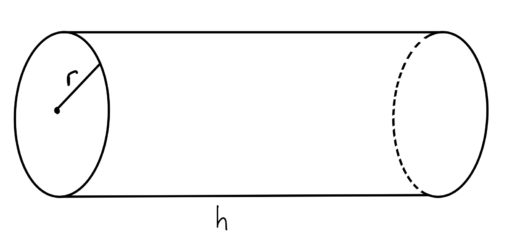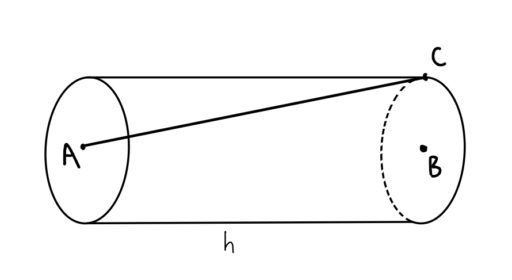The next 3D shape we shall look at is the circular cylinder (also called the “right circular cylinder” by the GRE for its right angles):

Circular Cylinder: A 3D shape that has a circle for both bases and a perpendicular line connecting the center of those bases.Now, it follows from the definition that a circular cylinder’s top and bottom circles will be the same size. So we useto denote the radius of either circle, and we useto denote the height of the cylinder. Without further ado, we present:

Volume of a Circular Cylinder: The volume of a right circular cylinder with a radius ofand a height ofisSurface Area of a Circular Cylinder: The surface area of a circular cylinder with a radius ofand a height ofisPractice Problems:

1. The cylinder below has a radius ofand a heightof 6. Find.2. The surface area of a right circular cylinder isand its radius is 2. What is the height of the cylinder?

3. The surface area of a cylinder is twice its volume. Its radius equals its height. What is the volume of the cylinder?